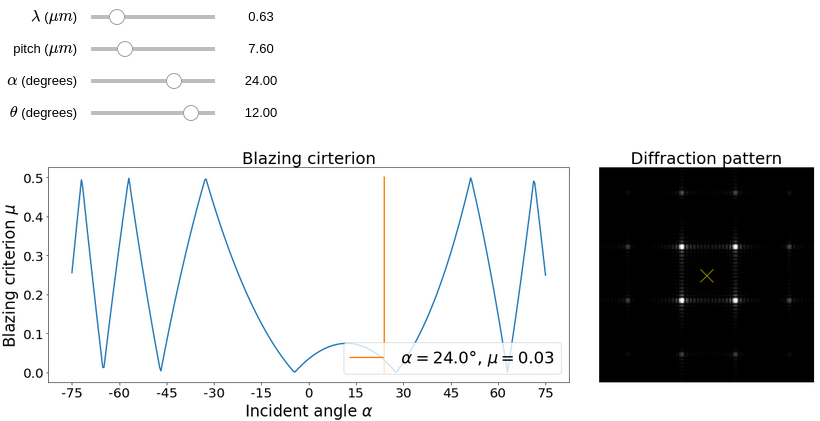## DMD diffraction toolI presented in this tutorial the diffraction effects occurring in a DMD setup. It corresponds to a blazed grating effect and depends on the wavelength $\lambda$, the pixel pitch $d$, the incident angle $\alpha$, and the angle of the micro-mirrors $\theta$. Here is a simple app (see source code on Github) to calculate the criterion for optimal diffraction efficiency and the aspect of the diffraction pattern in the far-field when illuminating the DMD with a plane wave of incident angle $\alpha$ with respect to the normal of the surface.

Click on "Show widget", it may take a minute to load.

#HIDDEN
# Written by Sebastien Popoff
# 29/10/2016
import matplotlib.pyplot as plt
import numpy as np
%matplotlib inline
import matplotlib as mpl
from matplotlib import rc
# rc('figure', figsize=(14,8))
from ipywidgets import interact, interact_manual, FloatSlider
#rc('text', usetex=True)

#HIDDEN
def mu_function(theta, d, _lambda):
## A simple criterion matching the diffraction angle and the reflection angle
_beta = lambda x: (2*theta-x)
return lambda x: 1.*d/_lambda *(np.sin(x)+np.sin(_beta(x)))
# when mu is an integer, we are at a blazing angle with a maximum of energy at the order along the optical axis
# whem mu is n+1/2, the enregy is spread over many diffraction orders not aligned with the optical axis

def get_mu(theta_1D, d, _lambda):

mu = mu_function(theta_1D, d, _lambda)
# test different incident angles
alpha_vec = np.linspace(-75,75,400)*np.pi/180
alpha_1D_vec = np.arctan(np.tan(alpha_vec)/np.sqrt(2))
criterion = np.abs(np.mod([mu(a) for a in alpha_1D_vec],1)-0.5)

return alpha_vec, criterion

#HIDDEN
def get_diffraction_pattern(_lambda, d, alpha, theta):

## A full numerical simulation of the Fourier plane for an all-on configuration
beta = 2*theta-alpha
N_mirrors = 15 # number of mirrors in each direction
gap = 2 # gap between pixels in micron
res = 10 # pixels per mirror (for numerical calculation)
Nx = N_mirrors*res

## Pixelate image function
f = np.ones([N_mirrors,N_mirrors]) # all-on configuration
## Phase slope due to incident and reflection angle
X,Y = np.meshgrid(np.arange(N_mirrors),np.arange(N_mirrors))
phi = np.exp((X-Y)*complex(0,1)*2*np.pi/_lambda*d*(np.sin(alpha)+np.sin(beta)))
## cell unit
Cell = np.zeros([res,res])
gpix = int(np.round(gap/(2.*d)*res))
Cell[gpix:res-gpix,gpix:res-gpix] = 1.
## Mirror image
MI = np.zeros([Nx,Nx],dtype='complex')
for i in range(N_mirrors):
for j in range(N_mirrors):
MI[i*res:(i+1)*res,j*res:(j+1)*res]= f[i,j]*phi[i,j]*Cell

## In the Fourier plane
coeff = 5
FP = np.fft.fftshift(np.fft.fft2(MI,s=[coeff*Nx,coeff*Nx]))
ROIsize = 300
ROI = 1

return FP[coeff*Nx//2-ROIsize//2:coeff*Nx//2+ROIsize//2,coeff*Nx//2-ROIsize//2:coeff*Nx//2+ROIsize//2]

def make_figure(_lambda, d, alpha, theta):

theta = theta/180*np.pi
alpha = alpha/180*np.pi
alpha_1D = np.arctan(np.tan(alpha)/np.sqrt(2))
theta_1D = np.arctan(np.tan(theta)/np.sqrt(2))

far_field = get_diffraction_pattern(_lambda, d, alpha_1D, theta_1D)
alpha_vec, mu_vec = get_mu(theta_1D, d, _lambda)
mu = mu_function(theta_1D, d, _lambda)

f, (a0, a1) = plt.subplots(1, 2, gridspec_kw={'width_ratios': [2, 1]}, figsize = (18,6))
a0.plot(alpha_vec*180/np.pi,mu_vec,linewidth = 2)
mu_alpha = np.abs(np.mod(mu(alpha_1D),1)-0.5)
label = fr' $\alpha = {alpha*180/np.pi:.1f}°$, $\mu = {mu_alpha:.2f}$'
a0.plot([alpha*180/np.pi,alpha*180/np.pi],[0.,0.5],label = label,linewidth = 2)
a0.set_xticks(np.arange(-75,75+15,15))
a0.set_xticklabels(np.arange(-75,75+15,15),fontsize = 20)
a0.set_yticks(np.arange(0,0.55,0.1))
a0.set_yticklabels([f'{x:.1f}' for x in np.arange(0,0.55,0.1)], fontsize = 20)
a0.set_title(r'Blazing cirterion', fontsize = 25)
a0.legend(fontsize = 25, loc='lower right')
a0.set_ylabel(r'Blazing criterion $\mu$', fontsize = 24)
a0.set_xlabel(r'Incident angle $\alpha$', fontsize = 24)

a1.imshow(
np.abs(far_field),
interpolation = 'None',
clim = [0,(np.max(np.abs(far_field)))/1.5],
cmap='gray'
)
a1.scatter(
far_field.shape//2,
far_field.shape//2,
s=400,
alpha = 0.5,
c='yellow',
marker='x'
)
a1.axis('off')
a1.set_title('Diffraction pattern', fontsize = 25)

f.tight_layout()

#HIDDEN
theta_slider = FloatSlider(
min = -20,
max = 20,
step = 1,
value = 12,
continuous_update=False,
description = r'$\theta$ (degrees)'
)
pitch_slider = FloatSlider(
min = 5,
max = 15,
step = 0.1,
value = 7.6,
continuous_update=False,
description = r'pitch ($\mu m$)'
)
lambda_slider = FloatSlider(
min = 0.3,
max = 2.,
step = 0.001,
value = 0.632,
continuous_update=False,
description = r'$\lambda$ ($\mu m$)'
)
alpha_slider = FloatSlider(
min = -75,
max = 75,
step = 1,
value = 24,
continuous_update=False,
description = r'$\alpha$ (degrees)'
)
interact(make_figure,
theta = theta_slider,
d = pitch_slider,
alpha = alpha_slider,
_lambda = lambda_slider);




Created by sebastien.popoff on 23/06/2020